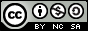- 目录 -

## 输入

3
7 4 7
2 50


## 输出

49


## 思路

• dp_max[i][j]:以第 i 个人为结尾，选择 j 个人所能获得的最大乘积

• dp_min[i][j]:以第 i 个人为结尾，选择 j 个人所能获得的最小乘积

$dp_{max}[i][j] = max(dp_{max}[i][j], max(dp_{max}[l][j - 1] * a[i], dp_{min}[l][j - 1] * a[i]))$

## 代码

  1 2 3 4 5 6 7 8 9 10 11 12 13 14 15 16 17 18 19 20 21 22 23 24 25 26 27 28 29 30 31 32 33 34 35  #include using namespace std; typedef long long ll; const ll inf = 1e18; const ll N = 50 + 10; ll a[N], dp_max[N][N], dp_min[N][N]; int main() { //freopen("in.txt", "r", stdin); ll n, k, d; scanf("%lld", &n); for (ll i = 1; i <= n; i++) { scanf("%lld", &a[i]); dp_max[i] = dp_min[i] = a[i]; } scanf("%lld%lld", &k, &d); for (ll i = 1; i <= n; i++) { for (ll j = 2; j <= k; j++) { if (i >= j) for (ll l = max(j - 1, i - d); l <= i - 1; l++) { dp_max[i][j] = max(dp_max[i][j], max(dp_max[l][j - 1] * a[i], dp_min[l][j - 1] * a[i])); dp_min[i][j] = min(dp_min[i][j], min(dp_max[l][j - 1] * a[i], dp_min[l][j - 1] * a[i])); } } } ll ans = -inf; for (ll i = k; i <= n; i++) ans = max(ans, dp_max[i][k]); printf("%lld\n", ans); return 0; }